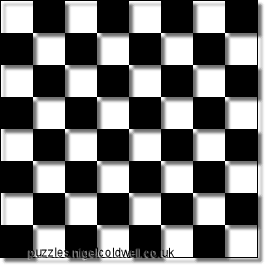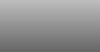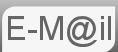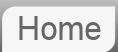# Puzzle #27: Number of Squares on a Chessboard

27. How many squares are there on a chessboard or chequerboard?? (the answer is not 64)

Can you extend your technique to calculate the number of rectangles on a chessboard?

Another puzzle that was e-mailed to me through this website. My instinct was that the answer was just a lot, but I thought about it and the solution is actually fairly simple...

The first thing is why the answer is not just 64...All the red squares in the above picture would count as valid squares, so we are asking how many squares of any dimension from 1x1 to 8x8 there are on a chess board.

The key is to think how many positions there are that each size of square can be located... A 2x2 square, for example, can, by virtue of it's size, be located in 7 locations horizontally and 7 locations vertically. ie in 49 different positions. A 7x7 square though can only fit in 2 positions vertically and 2 horizontally. Consider what's below...size horizontal positions vertical positions positions
1x1 8 8 64
2x2 7 7 49
3x3 6 6 36
4x4 5 5 25
5x5 4 4 16
6x6 3 3 9
7x7 2 2 4
8x8 1 1 1
total 204

In total there are 204 squares on a chessboard. This is the sum of the number of possible positions for all the squares of size 1x1 to 8x8.

## Formula For n x n Chessboard?

It's clear from the analysis above that the solution in the case of n x n is the sum of the squares from n2 to 12 that is to say n2 + (n-1)2 + (n-2)2 ... ... 22 + 12

Mathematically that is written as follows:The proof of the explicit solution is beyond the scope of this site, but if you want to look it up a mathematician would refer to it as 'the sum of the squares of the first n natural numbers.' The final answer is given by

n3/3 + n2/2 + n/6

## Can you extend your technique to calculate the number of rectangles on a chessboard?

Below are some examples of possible rectangles...All of the above examples would be vailid rectanges...

There is more than one way of solving this. But it makes sense to extend our technique from the squares problem first. The key to this is to think of each rectangle individually and consider the number of positions it can be located. For example a 3x7 rectangle can be located in 6 positions horizontally and 2 vertically. From this we can build a matrix of all the possible rectangles and sum.

 1296 Dimensions 1 2 3 4 5 6 7 8 Positions 8 7 6 5 4 3 2 1 1 8 64 56 48 40 32 24 16 8 2 7 56 49 42 35 28 21 14 7 3 6 48 42 36 30 24 18 12 6 4 5 40 35 30 25 20 15 10 5 5 4 32 28 24 20 16 12 8 4 6 3 24 21 18 15 12 9 6 3 7 2 16 14 12 10 8 6 4 2 8 1 8 7 6 5 4 3 2 1

In total then there are 1296 possible rectangles.

## Elegant approach to rectangles, consider the vertices and diagonals.I've been sent an innovative solution to the problem of the number of rectangles on a chessboard by Kalpit Dixit. This solution tackles the issue from a different approach. Rather than looking at specific sizes of rectangles and working out where they can be located we start at the other end and look at locations first.

The vertices are the intersections. For our chessboard there are 81 (9 x 9). A diagonal starting at one vertex and ending at another will uniquely describe a rectangle. In order to be a diagonal and not a vertical or horizontal line we may start anywhere but the end point must not have the same vertical or horizontal coordinate. As such there are 64 (8 x 8) possible end points.

There are therefore 81 x 64 = 5184 acceptable diagonals.

However, whilst each diagonal describes a unique rectangle, each rectangle does not describe a unique diagonal.We see trivially that each rectangle can be represented by 4 diagonals.

So our number of rectangles is given by 81 x 64 /4 = 1296

## n x n or n x m?

The n x n (eg. 9x9,) or n x m (eg 10x15,) problems can now be calculated. The number of vertices being given by (n + 1)2 and (n + 1).(m + 1) respectively. Hence the final solutions are as follows.

n x n: (n + 1)2 x n2 / 4
n x m: (n + 1) x (m + 1) x (n x m) / 4

Which can obviously be arranged into something more complicated.

## Rectangles in Maths Nomenclature

It's always my intention to explain the problems without formal maths nomenclature, with reasoning and common sense. But there is quite a neat solution here if you do know about combinations, as in permutations and combinations. Horizontally we are selecting 2 vertices from the 9 available. The order does not matter so it's combinations rather than permutations. And the same vertically. So the answer to the rectangle problem can be answered by:

9C29C2 = 362 = 1296

© Nigel Coldwell 2004 -  – The questions on this site may be reproduced without further permission, I do not claim copyright over them. The answers are mine and may not be reproduced without my expressed prior consent. Please inquire using the link at the top of the page. Secure version of this page.

PayPal
I always think it's arrogant to add a donate button, but it has been requested. If I help you get a job though, you could buy me a pint! - nigel